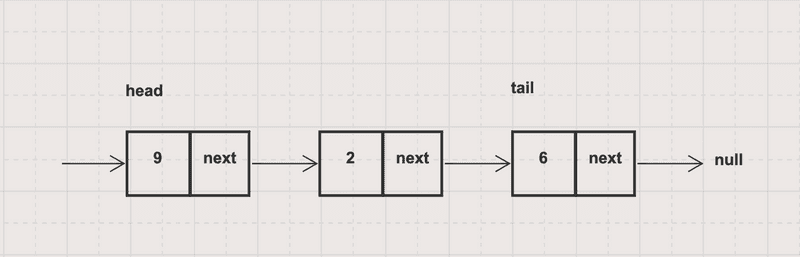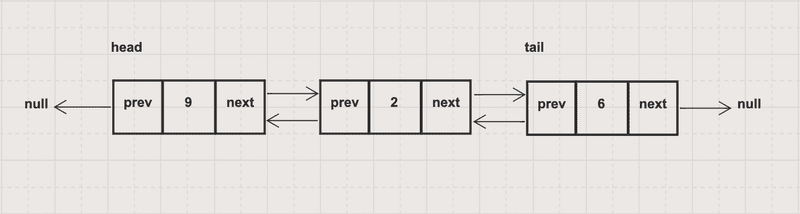# HelloKoding

Practical coding guides

Linked List is a linear data structure consisting of a collection of elements (nodes) which are stored in separated physical memory locations and linked to each other via pointer reference. Typically, there are two type of linked list: singly and doubly

Each node in a singly linked list comprises two parts, the data and the pointer which is linked to the next node. The next pointer of the tail node is linked to nullOperations and Time complexity

• Traversal O(N)
• Remove First O(1), Remove Last O(N)

``````package com.hellokoding.datastructure;

return;
}

Node newNode = new Node(data);

}

return;
}

while (currentNode.next != null) {
currentNode = currentNode.next;
}

currentNode.next = new Node(data);
}

public void removeFirst() {

}

public void removeLast() {

return;
}

while (currentNode.next.next != null) {
currentNode = currentNode.next;
}

currentNode.next = null;
}

public void traversal() {
while (currentNode != null) {
System.out.printf("%d ", currentNode.data);
currentNode = currentNode.next;
}

System.out.println();
}

public static void main(String[] args) {

}

static class Node {
public int data;
public Node next;

public Node(int data) {
this.data = data;
}
}
}
``````

Each node in a doubly linked list comprises three parts, the data and two pointers in which one is linked to previous node and the other is linked to next node. The previous pointer of the head node is linked to null while the next pointer of the tail node is linked to nullOperations and Time complexity

• Traversal O(N)
• Remove First O(1), Remove Last O(1)

``````package com.hellokoding.datastructure;

Node tail;

Node newNode = new Node(null, data, this.head);
if(this.tail == null) this.tail = this.head;
}

Node newNode = new Node(this.tail, data, null);
this.tail = newNode;
}

public void removeFirst() {

if (this.head == null) this.tail = null;
}

public void removeLast() {

this.tail = null;
return;
}

Node beforeTail = this.tail.previous;
beforeTail.next = null;
this.tail = beforeTail;
}

public void traversal() {
while (currentNode != null) {
System.out.printf("%d ", currentNode.data);
currentNode = currentNode.next;
}

System.out.println();
}

public static void main(String[] args) {

}

static class Node {
public int data;
public Node previous;
public Node next;

public Node(Node previous, int data, Node next) {
this.previous = previous;
if (previous != null) previous.next = this;

this.data = data;

this.next = next;
if (next != null) next.previous = this;
}
}
}
``````